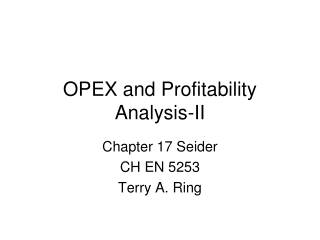# OPEX and Profitability Analysis-II - PowerPoint PPT PresentationDownload PresentationOPEX and Profitability Analysis-II

OPEX and Profitability Analysis-IIDownload Presentation## OPEX and Profitability Analysis-II

- - - - - - - - - - - - - - - - - - - - - - - - - - - E N D - - - - - - - - - - - - - - - - - - - - - - - - - - -
##### Presentation Transcript

1. OPEX and Profitability Analysis-II Chapter 17 Seider CH EN 5253 Terry A. Ring

2. Help On Costing Pumps Centrifugal, gear, etc. • Sizing Factor, S = Q*H0.5 • Q is the flow rate in Gal/min • H is the pump head • H=ΔP/ρg (pump change in P/liquid density) • CB=exp(A0+ A1(ln(S))+ A1(ln(S))2+ …) • Cp=FTypeFMat CB • There is no Fpressure term but if you had one it would use the outlet pressure • Motor Power, PMotor=Pp/(ηpumpηMotor) =QρgH/(ηpumpηMotor)

3. Measures of Profitability • Return on Investment (ROI) • ROI=annual earnings/capital investment • ROI > cost of capital (commercial interest rate, i) • Payback period (PBP) • Annualized Cost (CA) • Venture profit (VP) • Investor’s Rate of Return (IRR) • Discounted Cash Flow Rate of Return (DCFR) • Hard work to get this all together Same

4. Definitions of Profitability Measures

5. Total Capital Investment

6. How to determine ROI • Annual Sales revenue minus Costs (S-C) • Costs • Cost of feedstocks • Cost of Utilities • Cost of Labor and Maintenance • Cost of Overhead • Cost of Taxes and Insurance • Cost of Depreciation • Cost of sales force, R&D, Admin., Management Incentives

7. Utilities • Steam • Electricity • Cooling Water • Process Water • Boiler Water • Feed Stock water/Steam • Refrigeration and/or Chilled Brine • Fuels • Natural Gas • Fuel Oil • Coal • Waste Treatment • Stack Gas Cleanup • Waste Water Treatment • Area Scrubbers • Land fill cost for solid waste

8. Costs

9. Depreciation • Straight-line Depreciation • Equipment Lifetime – Plant = 12 yr • Constant % each year so that plant is totally written off at end of life time • 8% of Total Depreciable Capital, CTDC • What would be the depreciation % for 20 yr lifetime? • Other types of depreciation • Accelerated Cost Recovery System (ARCS) • Modified Accelerated Cost Recovery System (MARCS) for Taxes • Declining-Balance Method (DB) • Double Declining-Balance Method (DDB) • Others

10. More On Depreciation • Declining Balance Method (no salvage value) • d= range from 1/n to 2/n, typically use 1.5/n • Double Declining Balance Method (no salvage value) • d=2/n • Depreciation amount for year t, Dt=B*d*(1-d)t-1 • Book Value after year t, BVt-1 =B(1-d)t-1 • B=basis = CTDC • n= service life • t=year

11. More On Depreciation • ACRS – Federal Tax Law 1982 – 1986 • MACRS - Federal Tax Law in 1987 Accelerated Cost Recovery System

12. Taxes • (Local) Property Taxes • Based upon the value of the property • Used in Cost of Manufacturing • Severance Tax = 12.5% of extracted mineral’s net value • State Taxes • Very between states, Corporate tax in UT = 5% • Federal Income Tax for Business • Typically taken to be 35% of gross earnings 21%

13. More on Depletion (of natural resource) • Cost Depletion = Units recovered this year*Unit value • Unit Value = Cost to acquire resource/estimate of recoverable units • Percentage Depletion

14. Total Production Cost, C • Cost of Manufacturing minus general expenses • C=COM+General Expenses • General Expenses (see slide 7) • Selling expenses, R&D, Admin. (top management), Management Incentive Package

15. Costs

16. Cost of Manufacturing (COM) • Direct manufacturing costs • Feedstocks, Utilities, labor related to operations, maintenance • Operating overhead • Fixed costs • Property Tax, Insurance, Depreciation

17. Pre-tax (Gross) Earnings • Gross Earnings = Sales (S) – Total Production Cost (C) • Net Earnings(Profit) = (1-t) Gross Earnings • Tax (t) = State (UT=5%) + Federal taxes (34%) 21%

18. Working Capital, CWC Typically 15 % CTCI More Accurate Working Capital Calculation CWC=Cash Reserves+Inventory+accounts receivable-accounts payable • Cash reserves - 30 days of raw materials, utilities, operations, Maintenance, operating overhead, property taxes, insurance and depreciation 8.33% of COM • Inventories = 7 days of products at sales prices • Accounts receivable - 30 days at sales price 8.33% of annual sales • Accounts payable – 30 days of feedstocks at purchase price, 8.33% of annual feed stock costs

19. Definitions of Profitability Measures

20. Cost AnalysisEmissionEquipmentOptions1) Fix it/day2) \$1million in New Equipment

21. Selling Price for Given ROI • For a new product without an established market • Sale price may very • High Sales price • Assume ROI say 40% (This is a home run!!) • Back calculate the sales price. • Low Sales price • Set VP to Zero • Back calculate the sales price • (this is the same as ROI calc above when imin is 20%)

22. ROI is not good enough for cash poor companies • They use annual Cash Flow for decisions • Years of Plant construction CF = -fDCTDC-CWC-Cland • Years of Plant Operation CF = (1-t)(S-C)+D • Depreciation D=fDCTDC • fD = fraction of TDC which is depreciated that year

23. Plant 1 Cash Flow

24. Using Excel for Profitability Analysis, Cash Flow and OPEX • Steps to get ready • Get Chemical Prices • Get Cost of Utilities • Run Aspen/ProMax • Raw Material Flow Rate (kg/yr) • Production Rates (kg/y) • Utilities used (kg/y) • Determine installed cost of equipment (CAPEX) • Only then are you ready to use this spread sheet